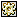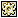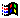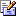Contents Articles Behaviors Books Director News Director Web Sites FAQ Games Mailing Lists News Groups Project Examples Reviews Software Tools Useful Web Sites Utilities Xtras

 Don't miss these#Count Down Timer

 Compatibilities:Required Xtras: Buddy API This item has not yet been rated

Author: BobKolk

This script will allow you to build a countdown timer that grabs the users system time and day and counts down to whatever time and day you wish. It was used in a screen saver to count down to an air date for a television program. This routine uses Buddy API (but you could code around it) and was only tested for the Windows platform, but I can"t see why it wouldn"t work on a Mac.

 on exitFrame     -- This is the Buddy API routines for grabbing the system date and time.   current_day=basystemtime("%j")   current_hour=basystemtime("%H")   current_min=basystemtime("%M")   current_sec=basystemtime("%S")   -- Set the target time in seconds   -- The 80 here is Day 80 of the year, the 20 is 8PM   target_time_sec=(80*24*60*60)+(20*60*60)     -- multiply the current day into seconds   -- the +30 at the end is a hack needed to fix an odd bug that would roll the minute counter   -- back at the 30 second mark (try eliminating the +30 (and hack routines below) and see what I mean).   current_time_sec=(current_day*24*60*60)+(current_hour*60*60)+(current_min*60)+current_sec+30     -- calculate the number of seconds between the target and current time   counter=target_time_sec-current_time_sec     -- checks to see if we have hit 00:00:00:00 (-30 due to the hack above)   if counter<=-30 then go to frame "thanks"   -- These calculations compute the seconds back into DD:HH:MM:SS     dis_sec=counter mod 60     counter=integer(counter/60)   dis_min=counter mod 60   counter=integer(counter/60)   dis_hrs=counter mod 24   counter=integer(counter/24)   dis_day=counter     -- code needed for the hack above (I know it"s yucky, but it works)   if dis_sec>30 then dis_sec_adj=dis_sec-30   if dis_sec<30 then dis_sec_adj=dis_sec+30   if dis_sec_adj=0 then dis_sec_adj="0"   -- pad the single digits with leading "0"     if dis_sec_adj<10 then dis_sec_adj="0"&string(dis_sec_adj)   if dis_min<10 then dis_min="0"&string(dis_min)   if dis_hrs<10 then dis_hrs="0"&string(dis_hrs)   if dis_day<10 then dis_day="0"&string(dis_day)   -- send the results out to the necessary text members     member(5).text=string(dis_day)   member(6).text=string(dis_hrs)   member(7).text=string(dis_min)   member(8).text=string(dis_sec_adj)     -- repeat (of course)   go to the frame end

 Contact MMI 36 South Court Sq Suite 300 Newnan, GA 30263 USASend e-mail